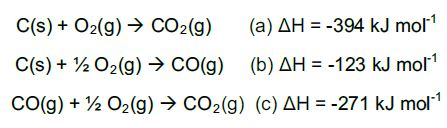Chemistry

# Hess’s Law

How can the enthalpy ΔH be discovered if the enthalpy changes for reactions are too difficult or impossible to determine experimentally?

If for example, the reaction is too slow or too fast or by-products are formed it is possible to then use Hess’s Law.

Definition: Hess’s Law states that the overall reaction enthalpy is the sum of the reaction enthalpies of each step of the reaction. This is a direct application of the First Law of Thermodynamics, which states that energy cannot be created or destroyed.

Finally, if the enthalpy ΔH for each step of the reaction is known, then these steps can be combined to give ΔH for the overall reaction.

There are two general methods of carrying out calculations based on Hess’s law: the pictorial method and the algebraic method.

The pictorial method requires a thermo-chemical cycle to show the energy changes between reactants and products in the different stages, whereas the algebraic method utilises only calculations.

In a chemical reaction, the energy change in converting reactants into products is the same, regardless of the route by which the chemical change occurs, as enthalpy is a state function. For example, the oxidation of carbon:Equation (a) is the sum of equations (b) and (c) and therefore

ΔHa = ΔHb + ΔHc.